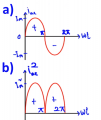# Determining the period of RMS sinusoidal waveform

#### cikalekli

Joined Dec 10, 2020
103
Here is the i (graph a) and i^2 graph (graph b) for the RMS calculation:My question is while calculating RMS value, should I decide to determine the period by looking a) or b) graph?
Because, while I'm looking for the a. graph (shown above), I guessed the period is 2pi
However, if I would consider the square of the graph (graph b), as far as I see that the period seems to be the only pi

So, is the period's value gonna be pi or 2pi?

I really wondered about it while I was studying RMS calculations...

#### MrAl

Joined Jun 17, 2014
10,064
Oh yeah, now I got it. It should be 2pi then
Actually in the strictest sense, it does not matter, even 10 cycles would work if you did it right. However, when calculating RMS you normally have to integrate and the simplest way to do that is to integrate over one half cycle which here would be just pi not 2*pi.
The reason this is true is because the longer the time you integrate over the longer the time is over which you are averaging (for the "Mean" part of RMS, and that means you are dividing by a larger number. Since you integrate over a longer time that increases the result of the integration and so when you divide by a large number you get the same result.
Try it both ways.

As a side issue...
Now when trying to determine the harmonic content that is different. That is because we almost always want to relate the harmonic number to the fundamental frequency, so we have to work over one full cycle which here would be 2*pi. I've seen that done over 1/2 cycle too (which would be just pi here) but that results in harmonics that are not related to the fundamental which would be very strange but maybe still possible. I cant think of any real life application for that but maybe someone else can.
An example would be trying to find out harmonics in a rectifier with a line frequency of 50 or 60 Hz. We would most likely want information related to those two frequencies not to 100 and 120 Hz. If we did that it would make the 2nd harmonic look like zero which cant be right.

Back to the RMS calculation...
Try the calculation for RMS over one half cycle, one full cycle, one and a half cycles, etc., and compare results.

Note that for more complex wave shapes it can be a little harder to determine the integration range because with waveforms with harmonic content the start and end times of the full cycle are not always apparent. This is especially true with waveforms with several unrelated harmonics mixed together. In that case it takes a little more work to figure out the full cycle, if there even is one in every case. We could look into this a bit more.
In waveforms that actually change with time it becomes necessary to go to some theoretical ideas about the type of waveform we are dealing with and the limits of how it can change.

Last edited: